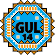General Utility Library for C++14  2.9
gul14/gcd_lcm.h

## Detailed Description

Greatest common divisor and least common multiple.

## Functions

template<typename IntTypeA , typename IntTypeB >
constexpr auto gul14::gcd (IntTypeA a, IntTypeB b)
Calculate the greatest common divisor of two integers using the Euclidean algorithm. More...

template<typename IntTypeA , typename IntTypeB >
constexpr auto gul14::lcm (IntTypeA a, IntTypeB b)
Calculate the least common multiple of two integers. More...

## ◆ gcd()

template<typename IntTypeA , typename IntTypeB >
 constexpr auto gul14::gcd ( IntTypeA a, IntTypeB b )
inlineconstexpr

Calculate the greatest common divisor of two integers using the Euclidean algorithm.

If both numbers are zero, the function returns zero. Otherwise, the result is a positive integer.

int greatest_common_divisor = gcd(10, 15); // returns 5
constexpr auto gcd(IntTypeA a, IntTypeB b)
Calculate the greatest common divisor of two integers using the Euclidean algorithm.
Definition: gcd_lcm.h:71
Returns
the least common multiple of the two numbers, represented as an integer type (`std::common_type_t<IntTypeA, IntTypeB>`) that both inputs can implicitly be converted to. If either a, b, or the result can not be represented by that type, the result is undefined.
Note
Unlike `std::gcd()` from C++17, the GUL14 version cannot be used with integers of different signedness. This avoids undefined behavior when mixing unsigned integers with negative signed values:
// C++17 gcd(): Undefined behavior - the common type is `unsigned int` and -5 has no
// representation in that type
// GUL14 gcd(): Does not compile
auto static_assertion_failure = gul14::gcd(10u, -5);
Since
GUL version 2.7

References gul14::abs().

Referenced by gul14::lcm().

## ◆ lcm()

template<typename IntTypeA , typename IntTypeB >
 constexpr auto gul14::lcm ( IntTypeA a, IntTypeB b )
inlineconstexpr

Calculate the least common multiple of two integers.

If both numbers are zero, the function returns zero. Otherwise, the result is a positive integer.

int least_common_multiple = lcm(10, 15); // returns 30
constexpr auto lcm(IntTypeA a, IntTypeB b)
Calculate the least common multiple of two integers.
Definition: gcd_lcm.h:125
Returns
the least common multiple of the two numbers, represented as an integer type (`std::common_type_t<IntTypeA, IntTypeB>`) that both inputs can implicitly be converted to. If either a, b, or the result can not be represented by that type, the result is undefined.
Note
Unlike `std::lcm()` from C++17, the GUL14 version cannot be used with integers of different signedness. This avoids undefined behavior when mixing unsigned integers with negative signed values:
// C++17 lcm(): Undefined behavior - the common type is `unsigned int` and -5 has no
// representation in that type